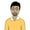# Learn React 18: Getting Started With StateState is used to manage information or data within React components. Unlike props, the state data can be changed throughout the lifetime of the component.

In this lesson from our free full-length beginner course on React 18, you'll learn how.

We have been creating React components for a while now. One thing that you might have noticed is that our React components only contained static data. However, you will also have to regularly deal with dynamic data when working with React.

We learned about props in a previous tutorial and how they pass data from parent components to a child. On the other hand, state is used to manage information or data within the components. Unlike props, the state data can be changed throughout the lifetime of the component.

## Updating Rendered Elements

Let's create a random number generator that gives us a new random number above a minimum value every two seconds. The code for the component would look like this:

 1 const rootElem = document.getElementById("root");  2 3 function RandomGenerator(props) {  4   5  let randomNumber = Math.floor(Math.random()*1000) + props.low;  6   7  setInterval(() => {  8  randomNumber = Math.floor(Math.random()*1000) + props.low;  9  }, 2000);  10   11  return (  12 
 13 

Random Number Generator

 14 

{randomNumber}

 15 
 16  );  17 }  18 19 let randomElem = (  20  <>  21   22   23 );  24 25 ReactDOM.createRoot(rootElem).render(randomElem); 

Our RandomGenerator component returns two heading elements wrapped inside a container div. We are using props to specify the lower limit for our random number. We use setInterval() to set a new value for randomNumber every two seconds.

You will probably expect this to output a new random number to be rendered on the webpage every two seconds. However, that doesn't actually happen. The element we generated from the component only represents what it would look like at a single specific point in time when it was generated.

One solution to our problem would be to re-render the element every two seconds using the code below.

 1 const rootElem = document.getElementById("root");  2 3 function RandomGenerator(props) {  4   5  let randomNumber = Math.floor(Math.random()*1000) + props.low;  6   7  return (  8 
 9 

Random Number Generator

 10 

{randomNumber}

 11 
 12  );  13 }  14 15 let randomElem = (  16  <>  17   18   19 );  20 21 function refresh() {  22  ReactDOM.createRoot(rootElem).render(randomElem);  23 }  24 25 refresh();  26 27 setInterval(refresh, 2000); 

As you can see in the demo, this technique works, and we get a new random number at regular intervals.

## Using State to Update Components

One problem with the code we wrote above is that our component is no longer independent. We are supposed to create encapsulated components which manage their own state and update the DOM if and when required. It also re-renders the whole component when the random number is generated, when it should only update the random number heading.

In this section, we will update our component to use state so that we don't have to make a render call from outside the component to get our number.

We have created our component as a function here. In earlier versions of React, you would have to convert the function component to a class in order to use state. However, we can now use hooks to get the same functionality.

Hooks are special functions that let you use React features like state within your function components. There are many hooks in React, but we will just discuss the useState hook here.

Calling useState() allows us to preserve some variables even after the function exits. In our example, we are keeping track of the value of the randomNumber variable. The only argument we pass to useState() is the initial state or value of our variable. It then returns a pair of values that have the current state and the function that updates the state.

 1 const rootElem = document.getElementById("root");  2 3 function RandomGenerator(props) {  4   5  const[randomNumber, setNumber] = React.useState(Math.floor(Math.random()*1000) + props.low);  6   7  setTimeout(() => setNumber(Math.floor(Math.random()*1000) + props.low), 2000);  8   9  return (  10 
 11 

Random Number Generator

 12 

{randomNumber}

 13 
 14  );  15 }  16 17 let randomElem = (  18  <>  19   20   21 );  22 23 ReactDOM.createRoot(rootElem).render(randomElem); 

We call our setNumber() function inside setTimeout every two seconds to get a new random number. There are a few things to note here.

It might seem counter-intuitive that we are using const for our randomNumber variable here, even though it is changing in value. The trick here is that the variable isn't actually changing in value within a single function call. Whenever we call setNumber() to update the value of the variable, it triggers a call to RandomGenerator() in order to re-render the component. The randomNumber variable will stay constant during each of these cycles, so that is why we used const. Also notice that we aren't using any assignment operator inside setNumber() to set a new value for randomNumber.

You might also be wondering why we didn't use setInterval() instead of setTimeout(). The reason is that using setInterval() will result in the creation of a new interval with every update. Using setTimeout() allows us to make an update once every two seconds.

## Final Thoughts

I hope this tutorial helped you understand how we can use state to manage dynamic data inside our components in React. For practice, try updating the above example so that it keeps track of the sum of all random numbers that we are generating.